Next: The uncertainty principle in Up: TIME-FREQUENCY RESOLUTION Previous: A misinterpretation of the

## Measuring the time-bandwidth product

Now examine Figure 2, which contains sampled Gaussian functions and their Fourier transforms. The Fourier transform of a Gaussian is well known to be another Gaussian function, as the plot confirms. I adjusted the width of each Gaussian so that the widths would be about equal in both domains. The Gaussians were sampled at various values of n, increasing in steps by a factor of 4. You can measure the width dropping by a factor of 2 at each step. For those of you who have already learned about the uncertainty principle, it may seem paradoxical that the function's width is dropping in both time and frequency domains.

 uncertain Figure 2 Sampled Gaussian functions and their Fourier transforms for vectors of length n = 16, 64, and 256.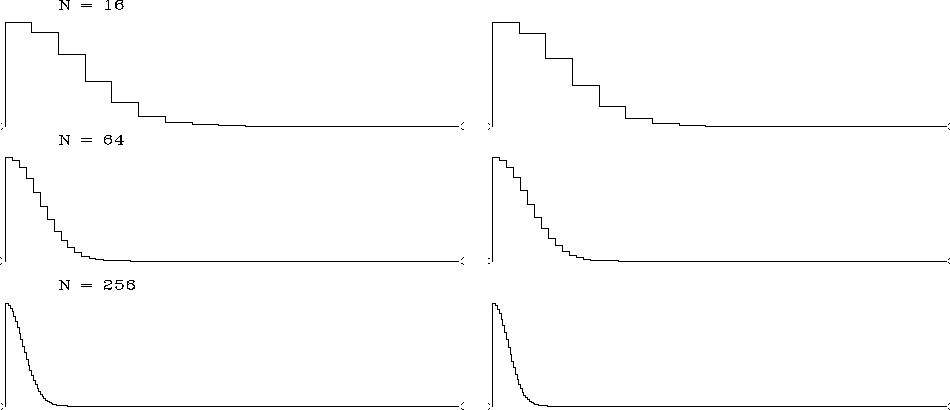The resolution of the paradox is that the physical length of the time axis or the frequency axis is varying as we change n (even though the plot length is scaled to a constant on the page). We need to associate a physical mesh with the computational mesh. A method of associating physical and computational meshes was described in chapteron page. In real physical space as well as in Fourier transform space, the object remains a constant size as the mesh is refined.

Let us read from Figure 2 values for the widths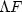and.On the top row, where N=16, I pick a width of about 4 points, and this seems to include about 90% of the area under the function. For this signal (with the widths roughly equal in both domains) it seems that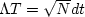and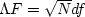.Using the relation between dt and df found in equation (), which says that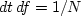,the product becomes= 1.

We could also confirm the inequality (1) by considering simple functions for which we know the analytic transforms--for example, an impulse function in time. Then, and the Fourier transform occupies the entire frequency band from minus to plus the Nyquist frequency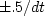Hz, i.e.,.Thus again, the product is= 1.Next: The uncertainty principle in Up: TIME-FREQUENCY RESOLUTION Previous: A misinterpretation of the
Stanford Exploration Project
10/21/1998